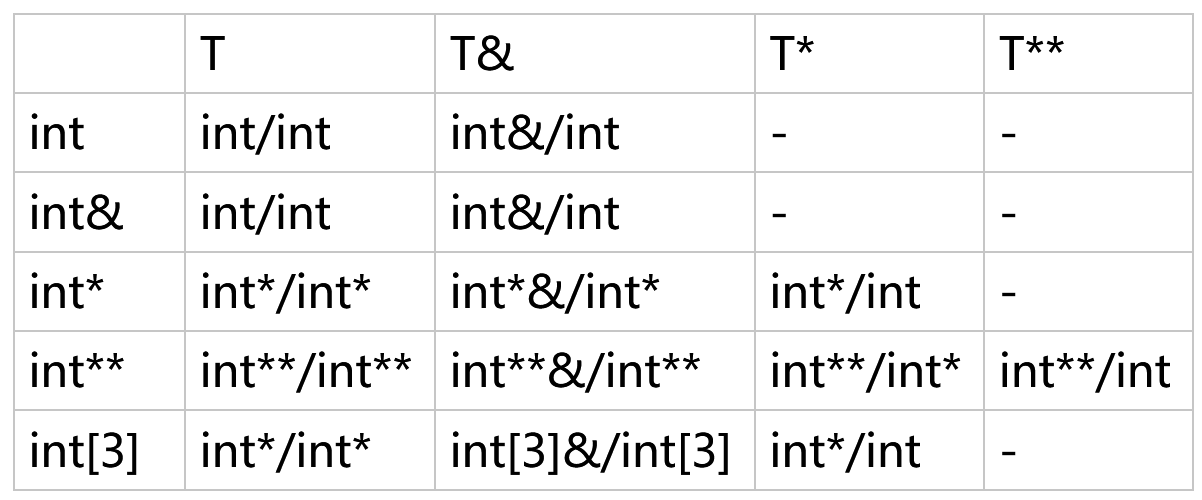# Effective Modern C++:Template and auto

The type deduction of Template and auto

The type deduction of template will ignore one level of reference and pointer

Example 1:

Input argument as reference

template<typename T>
void f(T& param);

int x = 27; // x is an int
const int cx = x; // cx is a const int
const int& rx = x; // rx is a reference to x as a const int
f(x);// T is int
f(cx);// T is const int, param's typeis const int&
f(rx);// T is const int, param's type is const int&


Example 2:

Input argument as pointer

template<typename T>
void f(T* param); // param isnow a pointer

int x = 27; // as before
const int *px = &x; // px is a ptr to x as a const int
f(&x); // T is int, param's typeis int*
f(px); // T is const int, param's type is const int*


Example 3:

Input argument as double reference, one will be ignored

template<typename T>
void f(T&& param); // param isnow a universal reference

int x = 27; // as before
const int cx = x; // as before
const int& rx = x; // as before
f(x); // x is lvalue, so T is int&, param's type is also int&
f(cx); // cx is lvalue, so T is const int&, param's type is also const int&
f(rx); // rx is lvalue, so T is const int&, param's type is also const int&
f(27); // 27 is rvalue, so T is int, param's type istherefore int&&


Example 4:

Input argument as value, cont and & will all be ignored

template<typename T>
void f(T param); // param is now passed by value

int x = 27; // as before
const int cx = x; // as before
const int& rx = x; // as before
f(x); // T's and param's types are both int
f(cx); // T's and param's types are again both int
f(rx); // T's and param's types are stillboth int


In the following table:

The type before / is the type of T

The type after / is the type of param-->

### WangxinI am algorithm engineer focused in computer vision, I know it will be more elegant to shut up and show my code, but I simply can't stop myself learning and explaining new things ...

### Gaussian Yolo V3 - get 3.5 more mAP and uncertainty with 1% more computation

#### Graph Optimization 4 - g2o introduction - GPS odometry

Published on November 18, 2018

#### Graph Optimization 3 - Optimization Update

Published on May 03, 2018